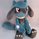# Exponential Regression Slope Annualized with R-squared Histogram2634 views
My other indicator shows the linear regression slope of the source. This one finds the exponential regression slope and optionally multiplies it by R-squared and optionally annualizes it. Multiplying by R-squared makes sure that the price movement was significant in order to avoid volatile movements that can throw off the slope value. Annualizing the exponential slope will let you see how much percentage you will make in a year if the price continues at its current pace.

The annualized number is the number of trading days in a year. This and the length might need adjusting for the extra bars that might be in futures or other markets. The number does not have to be a year. For example, it can be a month if you set the number to 20 or so trading days to find how much you would make in a month if price continues at its current pace, etc. This can also be used as an alternative to relative strength or rate of change .
Versionshinweise: Changed the default year from 250 to 252, not a big difference.
Changed the value to show percentage.
This indicator is like rate of change but lags in order to give less weight to the outliers or the prices that don't fit the exponential regression line.
Open-source Skript

Ganz im Sinne von TradingView hat der Autor dieses Skripts es als Open-Source veröffentlicht, damit Trader es verstehen und überprüfen können. Ein Hoch auf den Autor! Sie können es kostenlos verwenden, aber die Wiederverwendung dieses Codes in einer Publikation unterliegt den Hausregeln. Sie können das Skript den Favoriten hinzufügen, um es auf dem Chart zu verwenden.

Möchten Sie dieses Skript auf einem Chart verwenden?

## Kommentareliva4444
@liva4444, Chande Kroll R squared is the exact same indicator known as R squared used in other trading platforms such as thinkorswim. TV doesn't have its own R squared so I took the Chande Kroll one I found on TV that uses pow and correlation in it cause it looks clean and cut compared to having to write out all the math involved like the other platforms do. Not a math expert but I'd say it's the most official looking R squared I've seen imo so I assume this is the one Clenow meant.
AntwortenWhy do you call exp on the slope here?
annualized_slope = (pow(exp(slope),daysInYear) - 1) * 100

The slope is already exponential since you already call log on the prices.
nlPrice = log(src)

Isn't this just simpler?
annualized_slope = pow(1+slope,daysInYear) * 100
AntwortenSkyte
@Skyte, The slope needs to be converted back by applying the exp function. This gives the percentage change per bar in the slope. It is then annualized by raising it to the power of however many days in a year there are(or however many bars in whatever length of time you want to see it).

This indicator comes from Andreas Clenow's book Stocks on the Move. I converted what he had in his Excel picture and description of it into an indicator, he specifically uses the exp function on there for that step. I then concluded it to be most likely correct because it looks similar enough to rate of change. It's not really available anywhere to double check, but I think I got it right..
AntwortenSparkyFlary
@SparkyFlary, yes, I think you did the same calculation as Clenow but I don't think it's necessary, since like I said you already used log on the price and this already gives you percent:
"A regression model will have unit changes between the x and y variables, where a single unit change in x will coincide with a constant change in y. Taking the log of one or both variables will effectively change the case from a unit change to a percent change."

Here it's also explained and done without it: https://teddykoker.com/2019/05/momentum-strategy-from-stocks-on-the-move-in-python/

The reason I asked in the first place is just that I'm using a python screener and I use the formula without the exp() (to have it simpler and I guess more accurate because of less calculations) so if you decide to change it the numbers will be more alike^^, but I guess it doesn't really matter that much.

Oh and btw thanks for writing this indicator. In any case it's nice to see the Clenow results in TradingView.
AntwortenSkyte
@Skyte, you could cut out the annualized slope and just use slope, that could save a little extra step in the math. Doing this however "annualized_slope = (pow(exp(slope),daysInYear) - 1) * 100" gives a number that a human can understand and relate to by knowing how much the price is going up in the form of a percentage in a given daysInYear length in time. If you're somehow very constraint on the computation you could just leave the slope part but it probably wouldn't be as useful as an indicator on a chart. I'm not too well versed in python but you might want to double check their math on that return function they did, it looks like they were trying to annualize it so it should look similar to mine hopefully.
Antworten